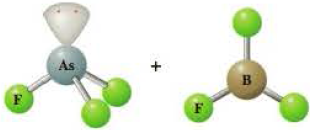# The following shows ball-and-stick models of the reactants in a Lewis acid–base reaction. Write the complete equation for the reaction, including the product. Identify each reactant as a Lewis acid or a Lewis base.### General Chemistry - Standalone boo...

11th Edition
Steven D. Gammon + 7 others
Publisher: Cengage Learning
ISBN: 9781305580343### General Chemistry - Standalone boo...

11th Edition
Steven D. Gammon + 7 others
Publisher: Cengage Learning
ISBN: 9781305580343

#### Solutions

Chapter
Section
Chapter 15, Problem 15.37QP
Textbook Problem

## The following shows ball-and-stick models of the reactants in a Lewis acid–base reaction.Write the complete equation for the reaction, including the product. Identify each reactant as a Lewis acid or a Lewis base.

Expert Solution
Interpretation Introduction

Interpretation:

The complete equation for the given reaction has to be written and each reactant as Lewis acid or a Lewis base has to be determined.

Concept introduction:

Lewis acid:

A species which can form a covalent bond by accepting electron pairs for the species is said to be Lewis acid.

Lewis base:

A species which can form a covalent bond by donating electron pairs for the species is said to be Lewis base.

### Explanation of Solution

The complete reaction for the given reaction can be represented as follows.

### Want to see the full answer?

Check out a sample textbook solution.See solution

### Want to see this answer and more?

Bartleby provides explanations to thousands of textbook problems written by our experts, many with advanced degrees!

See solution